You are currently offline. Some features of the site may not work correctly.

# Height of a polynomial

Known as: Length (disambiguation), Length of a polynomial, Polynomial height
In mathematics, the height and length of a polynomial P with complex coefficients are measures of its "size".
Wikipedia

## Papers overview

Semantic Scholar uses AI to extract papers important to this topic.
2016
2016
• 2016
• Corpus ID: 119155301
A positive integer k is a length of a polynomial if that polynomial factors into a product of k irreducible polynomials. We find… Expand
2015
2015
• 2015
• Corpus ID: 39371220
The Khovanov-Lauda-Rouquier (KLR) algebra arose out of attempts to cat- egorify quantum groups. Kleshchev and Ram proved a result… Expand
•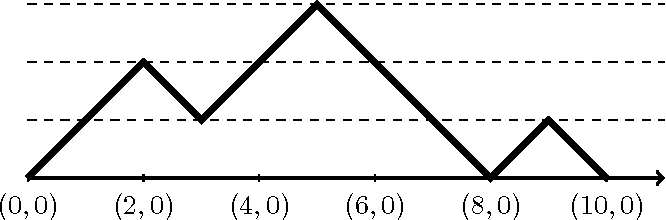•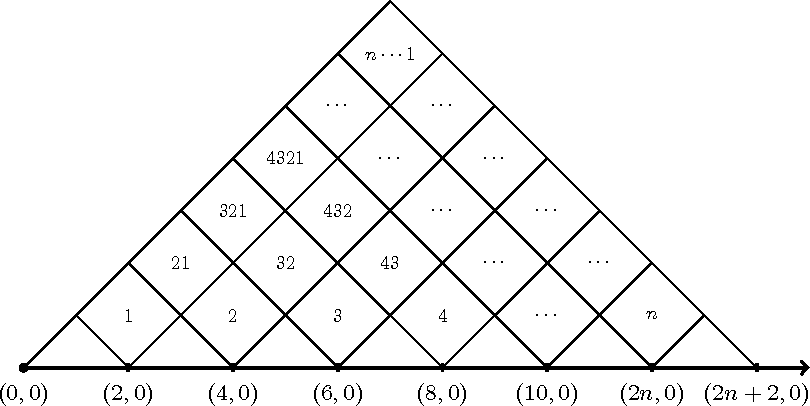•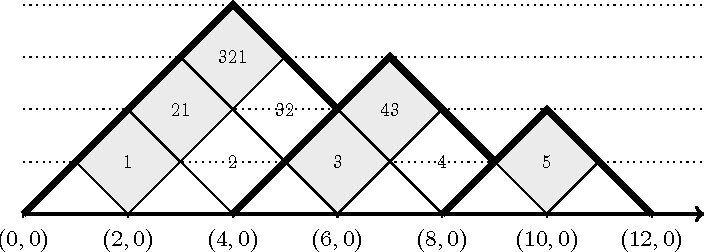•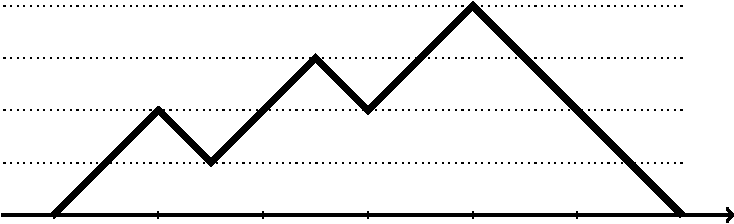2012
2012
We build a new theory for analyzing the coefficients of any cyclotomic polynomial by considering it as a gcd of simpler… Expand
2009
2008
2008
l(P ) = inf L(PG), l̂(P ) = min{l(P ), l(P ∗)}, where G runs through all monic polynomials in C[x]. This notation is consistent… Expand
2006
2006
• Math. Comput.
• 2006
• Corpus ID: 10717321
Let N*(m) be the minimal length of a polynomial with ±1 coefficients divisible by (x-1) m . Byrnes noted that N*(m) < 2 m for… Expand
2002
2002
It is shown that the methods established in [HKN3] can be effectively used to study polynomial cycles in certain rings. We shall… Expand
1993
1993
• Comput. Aided Geom. Des.
• 1993
• Corpus ID: 205070624
Abstract Sufficient and necessary conditions for the arc length of a polynomial parametric curve to be an algebraic function of… Expand
•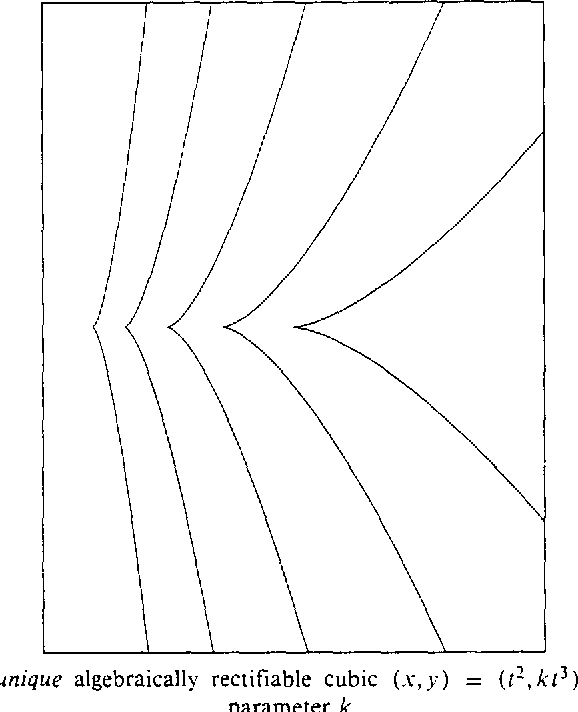•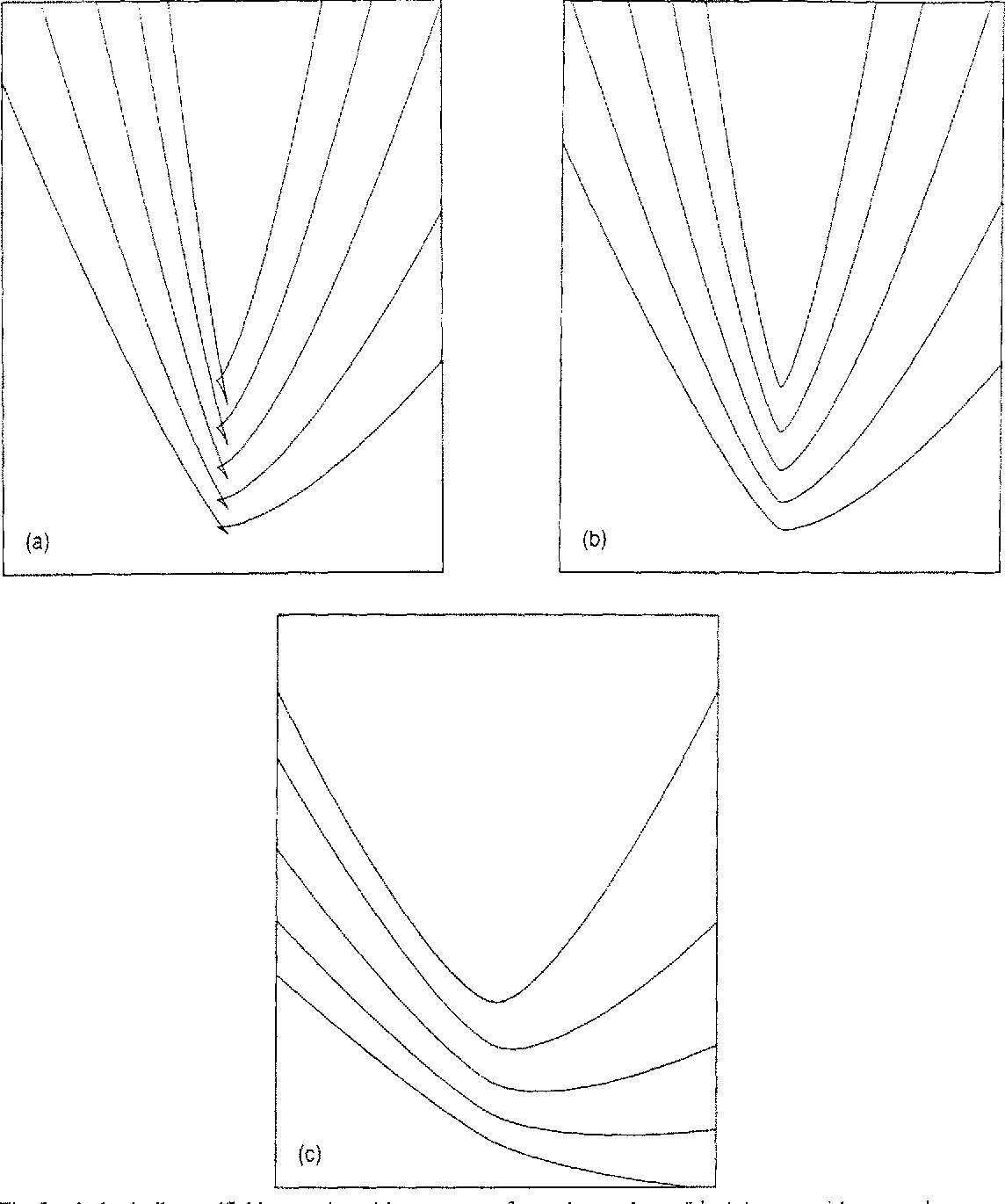•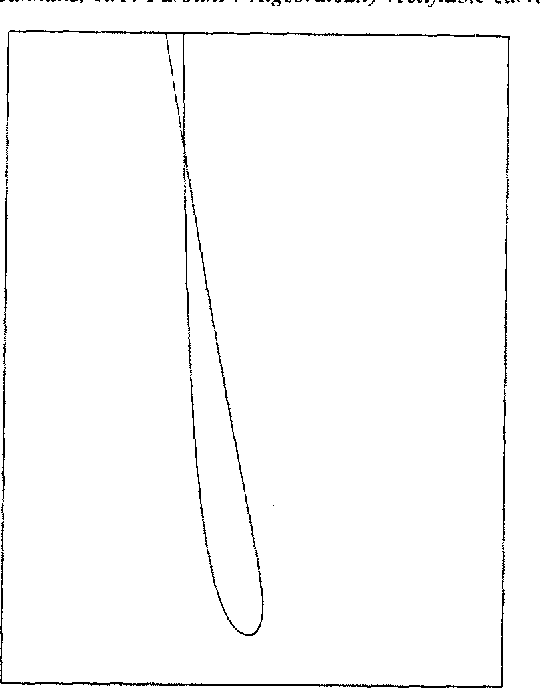•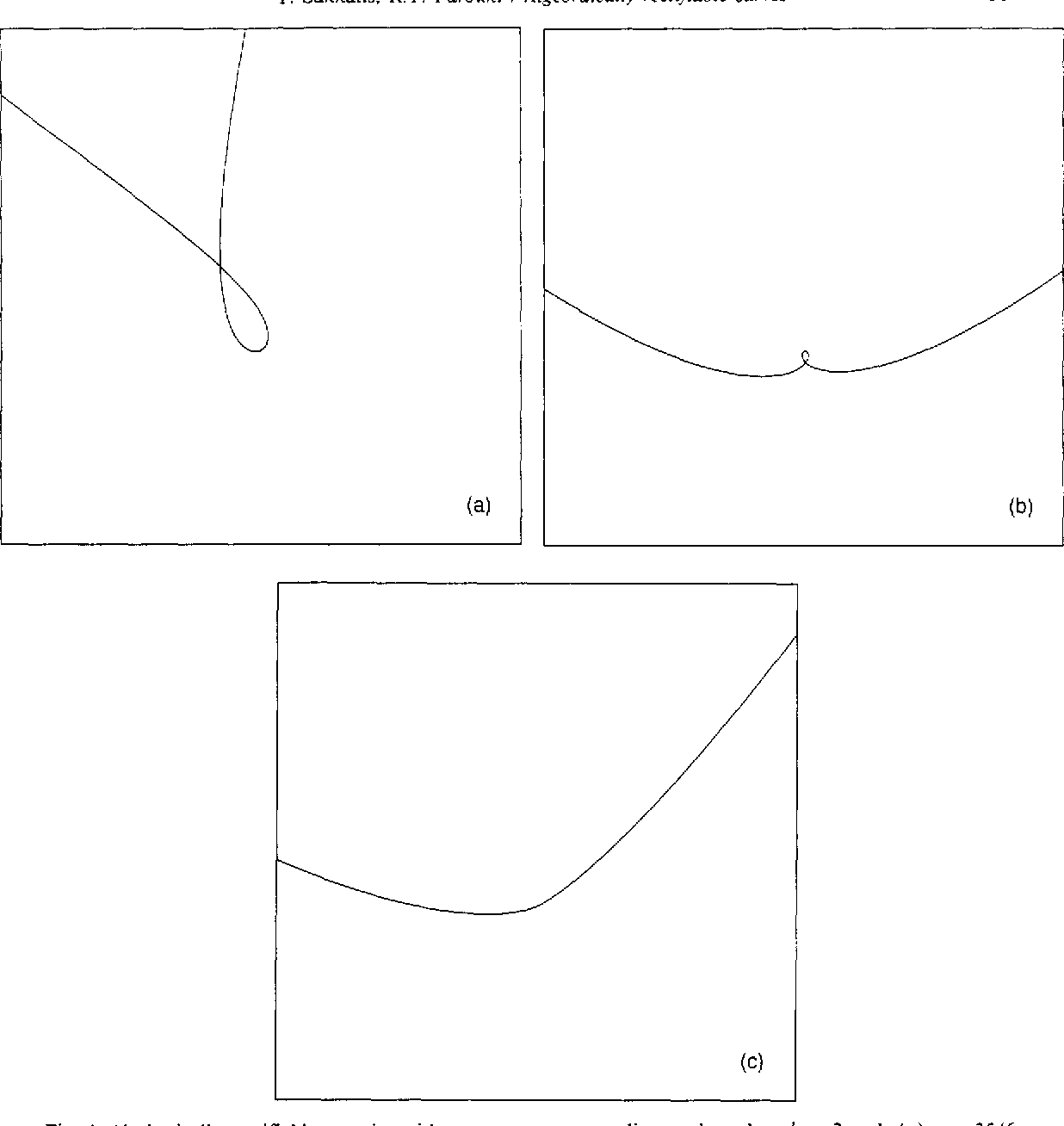1981
1981
Syntactical graphs are representations of derivations of arbitrary grammars. The height of syntactical graphs is introduced here… Expand